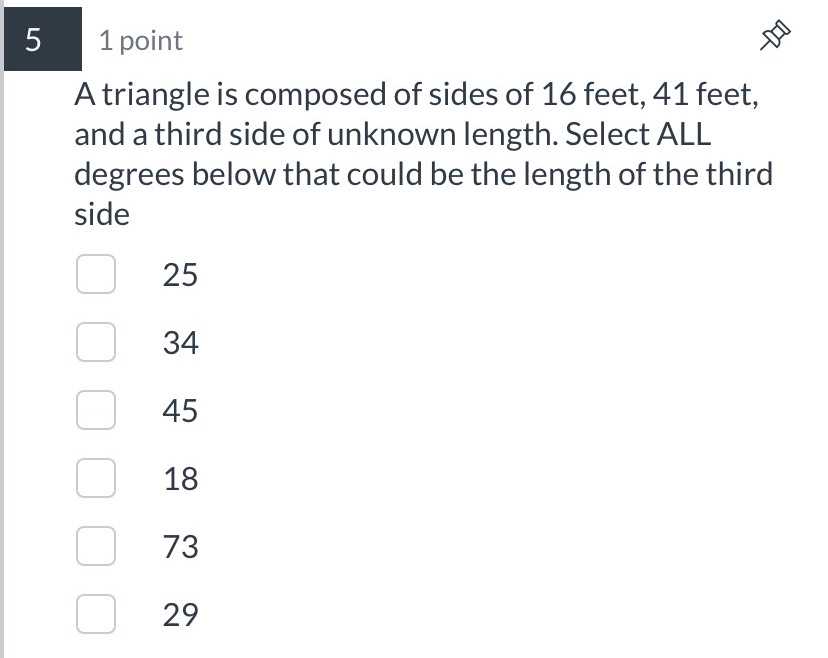### Still have math questions?

Trigonometry
QuestionA triangle is composed of sides of $$16$$ feet, $$41$$ feet, and a third side of unknown length. Select ALL degrees below that could be the length of the third side

$$25$$

$$34$$

$$45$$

$$18$$

$$73$$

$$29$$

$$34,45,29$$